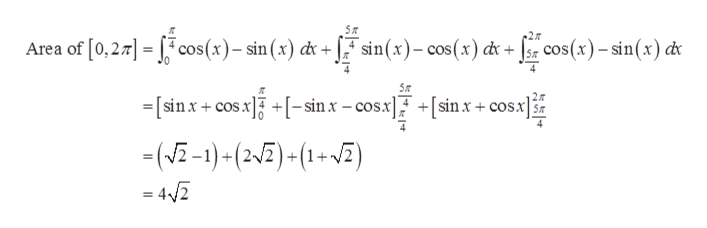# Find the area of the region R bounded by f(x)= sinx, g(x)=cosx, x=0 and x=20pi

Question
30 views

Find the area of the region R bounded by f(x)= sinx, g(x)=cosx, x=0 and x=20pi

check_circle

Step 1

Given, functions and interval

Step 2

Area bounded by curves is

Step 3

Area for first cycle i....help_outlineImage Transcriptionclose5T Area of [0,27 cos(x)-sin(x) d+ sin(x)-cos(x) dr cos(x)-sin(x) d [sinx+cosx -sinx -cosx sinx+ cosx -(-1)-(2)-(1-A) 4/2 fullscreen

### Want to see the full answer?

See Solution

#### Want to see this answer and more?

Solutions are written by subject experts who are available 24/7. Questions are typically answered within 1 hour.*

See Solution
*Response times may vary by subject and question.
Tagged in

### Integration